# Thresholding Images

This is a package for thresholding images. Let’s load it and some friends.

library(autothresholdr)
library(EBImage)
library(magrittr)

We’ll be using the image that comes with the package:

img <- imageData(readImage(system.file("extdata", "eg.tif",
package = "autothresholdr"),
as.is = TRUE))
display(normalize(img), method = "r")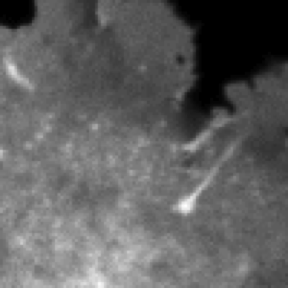It’s a bit of a cell, the black part is where the cell is not. The threshold is supposed to tell us what is dark (not cell) and what is bright (cell). By playing around, we see that something like 4 is a good value.

display(img > 4, method = "r")But what if we have many images and we don’t want to play around, we want a method of calculating the threshold automatically. http://imagej.net/Auto_Threshold gives many such methods and they are provided to you in R via this package. Go to that webpage for a nice comparison of the methods.

The function auto_thresh finds the threshold, auto_thresh_mask gets the mask (an array with a TRUE for elements exceeding the threshold and FALSE elsewhere) and auto_thresh_apply_mask applies the mask to the original image by setting the elements that don’t exceed the threshold to NA.

Let’s see each with Huang thresholding.

auto_thresh(img, "huang")
##  5
auto_thresh_mask(img, "huang") %>% display(method = "r")auto_thresh_apply_mask(img, "huang") %>% normalize %>%  display(method = "r")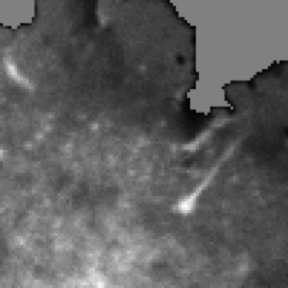In this last image, the NA pixels are greyed.

## Stacks of Images

We can do the same thing with an image stack.

img <- imageData(readImage(system.file("extdata", "50.tif",
package = "autothresholdr"),
as.is = TRUE))
display(normalize(img), method = "r")
## Only the first frame of the image stack is displayed.
## To display all frames use 'all = TRUE'.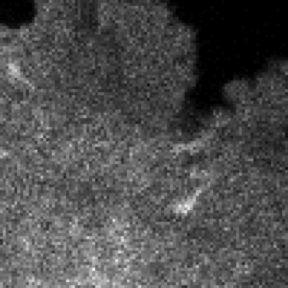mean_stack_thresh(img, "tri") %>% normalize %>%  display(method = "r")
## Only the first frame of the image stack is displayed.
## To display all frames use 'all = TRUE'.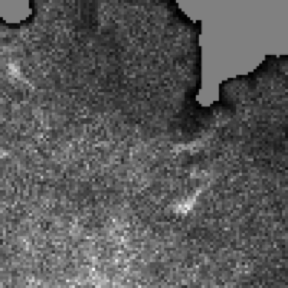med_stack_thresh(img, "tri") %>% normalize %>%  display(method = "r")
## Only the first frame of the image stack is displayed.
## To display all frames use 'all = TRUE'.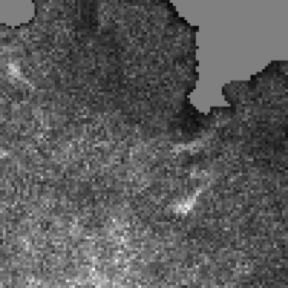These are indeed (on close inspection) slightly different.